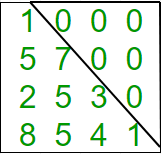GeeksforGeeks App
Open AppBrowser
Continue

# Php Program to check if matrix is lower triangular

Given a square matrix and the task is to check the matrix is in lower triangular form or not. A square matrix is called lower triangular if all the entries above the main diagonal are zero.Examples:

```Input : mat = {{1, 0, 0, 0},
{1, 4, 0, 0},
{4, 6, 2, 0},
{0, 4, 7, 6}};
Output : Matrix is in lower triangular form.

Input : mat = {{1, 0, 0, 0},
{4, 3, 0, 1},
{7, 9, 2, 0},
{8, 5, 3, 6}};
Output : Matrix is not in lower triangular form.```

## PHP

 ``

Output:

`Yes`

Time Complexity: O(n2), where n represents the number of rows and columns of the given matrix.
Auxiliary Space: O(1), no extra space is required, so it is a constant.

Please refer complete article on Program to check if matrix is lower triangular for more details!

My Personal Notes arrow_drop_up